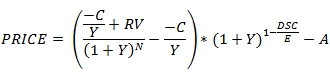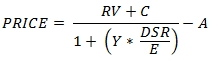SQL Server PRICE function

PRICE

Updated: 05 May 2014

Use PRICE to calculate the price for a security that pays periodic interest and has a par value of 100. The formula for price with more than one coupon period to redemption is:Where
C = 100 * coupon rate / frequency
Y = yield / frequency
RV = redemption value
DSC = number of days from settlement to coupon
N = the number of coupons between the settlement date and the maturity date
E = the number of days in the current coupon period
A = C * accrued days / E
When the settlement date is greater than or equal to the last coupon date, the formula for price is:Where
C = 100 * coupon rate / frequency
Y = yield / frequency
RV = redemption value
DSR = number of days from settlement to redemption
E = the number of days in the current coupon period
A = C * accrued days / E
Syntax
SELECT [westclintech].[wct].[PRICE] (
<@Settlement, datetime,>
,<@Maturity, datetime,>
,<@Rate, float,>
,<@Yld, float,>
,<@Redemption, float,>
,<@Frequency, float,>
,<@Basis, nvarchar(4000),>)
Arguments
@Settlement
the settlement date of the security. @Settlement is an expression that returns a datetime or smalldatetime value, or a character string in date format.
@Maturity
the maturity date of the security. @Maturity is an expression that returns a datetime or smalldatetime value, or a character string in date format.
@Rate
the security’s annual coupon rate. @Rate is an expression of type float or of a type that can be implicitly converted to float.
@Yld
the security’s annual yield. @Yld is an expression of type float or of a type that can be implicitly converted to float.
@Redemption
the security’s redemption value per 100 face value. @Redemption is an expression of type float or of a type that can be implicitly converted to float.
@Frequency
the number of coupon payments per year. For annual payments, @Frequency = 1; for semi-annual, @Frequency = 2; for quarterly, @Frequency = 4; for bimonthly @Frequency = 6; for monthly, @Frequency = 12. For bonds with @Basis = 'A/364' or 9, you can enter 364 for payments made every 52 weeks, 182 for payments made every 26 weeks, 91 for payments made every 13 weeks, 28 for payments made every 4 weeks, 14 for payments made every 2 weeks, and 7 for weekly payments. @Frequency is an expression of type float or of a type that can be implicitly converted to float.
@Basis
is the type of day count to use. @Basis is an expression of the character string data type category.

 @Basis Day count basis 0, 'BOND' US (NASD) 30/360 1, 'ACTUAL' Actual/Actual 2, 'A360' Actual/360 3, 'A365' Actual/365 4, '30E/360 (ISDA)', '30E/360', 'ISDA', '30E/360 ISDA', 'EBOND' European 30/360 5, '30/360', '30/360 ISDA', 'GERMAN' 30/360 ISDA 6, 'NL/ACT' No Leap Year/ACT 7, 'NL/365' No Leap Year /365 8, 'NL/360' No Leap Year /360 9, 'A/364' Actual/364 10, 'BOND NON-EOM' US (NASD) 30/360 non-end-of-month 11, 'ACTUAL NON-EOM' Actual/Actual non-end-of-month 12, 'A360 NON-EOM' Actual/360 non-end-of-month 13, 'A365 NON-EOM' Actual/365 non-end-of-month 14, '30E/360 NON-EOM', '30E/360 ICMA NON-EOM', 'EBOND NON-EOM' European 30/360 non-end-of-month 15, '30/360 NON-EOM', '30/360 ISDA NON-EOM', 'GERMAN NON-EOM' 30/360 ISDA non-end-of-month 16, 'NL/ACT NON-EOM' No Leap Year/ACT non-end-of-month 17, 'NL/365 NON-EOM' No Leap Year/365 non-end-of-month 18, 'NL/360 NON-EOM' No Leap Year/360 non-end-of-month 19, 'A/364 NON-EOM' Actual/364 non-end-of-month
Return Type
float
Remarks
·         If @Settlement is NULL then @Settlement = GETDATE().
·         If @Maturity is NULL then @Maturity = GETDATE().
·         If @Rate is NULL then @Rate = 0.
·         If @Yield is NULL then @Yield = 0.
·         If @Redemption is NULL then @Redemption = 100.
·         If @Frequency is NULL then @Frequency = 2.
·         If @Basis is NULL then @Basis = 0.
·         If @Frequency is any number other than 1, 2, 4, 6 or 12, or for @Basis = 'A/364' any number other than 1, 2, 4, 6, or 12 as well as 7, 14, 28, 91, 182, or 364 PRICE returns an error.
·         If @Basis is invalid (see above list), PRICE returns an error.
Examples
In this example we calculate the price for a bond maturing on 2034-06-15. The settlement date is 2014-05-01, the yield is 2.76%, the coupon rate is 2.50%, the redemption value is 100, the coupon is paid twice-yearly, and the basis code is 1.
SELECT wct.PRICE(
'2014-05-01',  --@Settlement
'2034-06-15',  --@Maturity
0.025,         --@Rate
0.0276,        --@Yield
100,           --@Redemption
2,             --@Frequency
1              --@Basis
) as PRICE

This produces the following result.
PRICE
----------------------
96.0043799057024

In this example, we calculate the price of a zero-coupon bond.
SELECT wct.PRICE(
'2014-05-01',  --@Settlement
'2044-06-15',  --@Maturity
0.00,          --@Rate
0.0301,        --@Yield
100,           --@Redemption
2,             --@Frequency
1              --@Basis
) as PRICE

This produces the following result.
PRICE
----------------------
40.6583576113141

In this example we calculate the price of a bond settling in the final coupon period.
SELECT wct.PRICE(
'2014-05-01',  --@Settlement
'2014-07-15',  --@Maturity
0.0190,        --@Rate
0.0005,        --@Yield
100,           --@Redemption
2,             --@Frequency
0              --@Basis
) as PRICE

This produces the following result.
PRICE
----------------------
100.380181205142

Here we calculate the price of a bond maturing on the 30th of September 2034, with semi-annual coupons payable on March 30th and September 30th.
SELECT wct.PRICE(
'2014-05-01',  --@Settlement
'2034-09-30',  --@Maturity
0.0257,        --@Rate
0.0269,        --@Yield
100,           --@Redemption
2,             --@Frequency
11             --@Basis
) as PRICE

This produces the following result.
PRICE
----------------------
98.1232907936385

Here's an example of the price calculation with a negative yield.
SELECT wct.PRICE(
'2014-05-01',  --@Settlement
'2014-09-30',  --@Maturity
0.0257,        --@Rate
-0.046219,     --@Yield
98,            --@Redemption
2,             --@Frequency
0              --@Basis
) as PRICE

This produces the following result.
PRICE
----------------------
101.000010706758

This is an example of a bond paying interest every 26 weeks.
SELECT wct.PRICE(
'2014-10-01',     --@Settlement
'2023-03-13',     --@Maturity
0.1250,           --@Rate
0.1100,           --@Yield
100,              --@Redemption
182,              --@Frequency
9                 --@Basis
) as PRICE
This produces the following result.
PRICE
----------------------
108.126105929164

SupportCopyright 2008-2022 Westclintech LLC         Privacy Policy        Terms of Service